# Chapter 22 , BJT Biasing and Stabilization¶

## Example 22.1 , Page Number 527¶

In :
import math
import numpy
%matplotlib inline
from matplotlib.pyplot import plot,title,xlabel,ylabel

#Variables

VBB = 10.0                    #Base Voltage (in volts)
RB = 47.0                     #Base Resistance (in kilo-ohm)
VCC = 20.0                    #Voltage Source (in volts)
RC = 10.0                     #Collector Resistance (in kilo-ohm)
beta = 100.0                  #Common-Emitter current gain

#Calculation

ICsat = VCC / RC              #Saturation current (in milli-Ampere)
VCEcutoff = VCC               #Cutoff voltage (in volts)

#Result

print "The value of saturation current is ",ICsat," mA.\nThe value of cut-off voltage is ",VCEcutoff," V."

#Graph

x = numpy.linspace(0,20,100)
plot(x,x/10)
xlabel("Collector-to-emitter voltage VCE (V)")
ylabel("Collector current IC (mA)")

The value of saturation current is  2.0  mA.
The value of cut-off voltage is  20.0  V.

Out:
<matplotlib.text.Text at 0x60d98f0>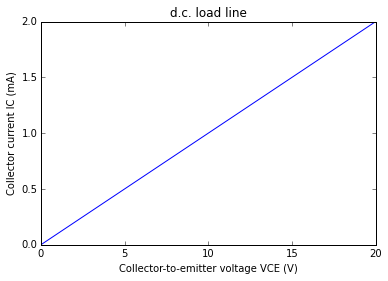## Example 22.2 , Page Number 528¶

In :
import math
import numpy
%matplotlib inline
from matplotlib.pyplot import plot,title,xlabel,ylabel,annotate

#Variables

VCC = 20.0                    #Source voltage (in volts)
RC = 300.0                    #Collector resistance (in ohm)
VBB = 10.0                    #Base voltage (in volts)
RB = 50.0                     #Base Resistance (in kilo-ohm)
beta = 200.0                  #Common-emittter current gain

#Calculation

ICsat = VCC / RC              #Saturation current (in Ampere)
VCEcutoff = VCC               #Cutoff voltage (in volts)
#Using kirchoff's voltage law
IB = (VBB - 0.7) / RB         #Base current (in milli-Ampere)
IC = beta * IB                #Collector current (in milli-Ampere)
VCE = VCC - IC * RC * 10**-3  #Collector-to-emitter voltage (in volts)

#Result

print "Q-points corresponds to ",VCE," V and ",IC," mA."

#Graph

x = numpy.linspace(0,20,100)
plot(x,66.7 - 66.7/20 * x)
xlabel("Collector-to-emitter voltage VCE (V)")
ylabel("Collector current IC (mA)")
annotate("Q",xy=(8.84,37.2))
annotate("66.7",xy=(0,66.7))
annotate("37.2",xy=(0,37.2))
annotate("8.84",xy=(8.84,0))

Q-points corresponds to  8.84  V and  37.2  mA.

Out:
<matplotlib.text.Annotation at 0x620f450>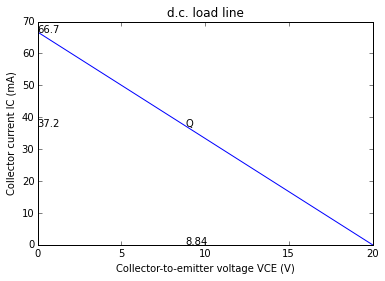## Example 22.3 , Page Number 533¶

In :
import math

#Variables

VCC = 25.0                    #Source voltage (in volts)
RC = 820.0                    #Collector Resistance (in ohm)
RB = 180.0                    #Base Resistance (in kilo-ohm)
beta = 80.0                   #Common-Emitter current gain

#Calculation

IB = VCC / RB                 #Base current (in milli-Ampere)
IC = beta * IB                #Collector current (in milli-Ampere)
VCE = VCC - IC * RC * 10**-3  #Collector-to-Emitter voltage (in volts)

#Result

print "The value of base current is ",round(IB,2)," mA.\nThe value of Collector current is ",round(IC,2)," mA.\nTHe value of Collector-to-Emitter voltage is ",round(VCE,2)," V."

#Slight variation in answers due to higher precision.

The value of base current is  0.14  mA.
The value of Collector current is  11.11  mA.
THe value of Collector-to-Emitter voltage is  15.89  V.


## Example 22.4 , Page Number 534¶

In :
#Variables

VBB = 2.7                       #Base voltage (in Volts)
RB = 40.0                       #Base resistance (in kilo-ohm)
VCC = 10.0                      #Supply voltage (in volts)
RC = 2.5                        #Collector resistance (in kilo-ohm)
VBE = 0.7                       #Emitter-to-base voltage (in volts)
beta = 100.0                    #Current gain

#Calculation

IB = (VBB - VBE)/RB             #Base current (in milli-Ampere)
IC = beta * IB

#Result

print "The base current is ",IB," mA."
print "The collector current is ",IC," mA."

The base current is  0.05  mA.
The collector current is  5.0  mA.


## Example 22.5 , Page Number 534¶

In :
import math

#Variables

VCC = 5.0                      #Source voltage (in volts)
RC = 5.0                       #Collector resistance (in kilo-ohm)
VBB = 5.0                      #Base voltage (in volts)
RB = 100.0                     #Base Resistance (in kilo-ohm)
VBE = 0.7                      #Emitter-to-Base Voltage (in volts)
beta = 30.0                    #Common-Emitter current gain

#Calculation

IB = (VBB - VBE)/RB            #Base Current (in milli-Ampere)
IC = beta * IB                 #Collector Current (in milli-Ampere)
IC1 = VCC / RC                 #Collector Current (in milli-Ampere)

#Result

print "The value of collector current is for operation in saturation region is ",IC1," mA.\nSince ",IC," mA is greater than ",IC1," mA , therefore it will operate in saturation region."

The value of collector current is for operation in saturation region is  1.0  mA.
Since  1.29  mA is greater than  1.0  mA , therefore it will operate in saturation region.


## Example 22.6 , Page Number 535¶

In :
import math

#Variables

VCC = 12.0                     #Source voltage (in volts)
RC = 330.0                     #Collector resistance (in ohm)
IB = 0.3                       #Base current (in milli-Ampere)
beta = 100.0                   #Common-emitter current gain

#Calculation

RB = VCC / IB                  #Resistance (in kilo-ohm)
S = 1 + beta                   #Stability factor
IC = beta * IB                 #Collector current (in milli-Ampere)
VCE = VCC - IC * RC * 10**-3   #Collector-Ground voltage (in volts)

#Result

print "Stability factor for the fixed bias circuit is ",S,".\nThe voltage between the collector and the ground is ",VCE," V."

Stability factor for the fixed bias circuit is  101.0 .
The voltage between the collector and the ground is  2.1  V.


## Example 22.7 , Page Number 536¶

In :
import math

#Variables

VCC = 20.0                      #Source voltage (in volts)
RC = 2.0                        #Collector resistance (in kilo-ohm)
RB = 400.0                      #Base Resistance (in kilo-ohm)
beta = 100.0                    #Common-Emitter current gain
RE = 1.0                        #Emitter Resistance (in kilo-ohm)

#Calculation

IB = VCC / (RB + beta * RE)     #Base current (in milli-Ampere)
IC = beta * IB                  #Collector Current (in milli-Ampere)
VCE = VCC - IC * (RC + RE)      #Collector-to-Emitter Voltage (volts)

#Result

print "VCE of the transistor is ",VCE," V.\nVCC of the transistor is ",VCC," V.\nIB of the transistor is ",IB," mA.\nIC of transistor is ",IC," mA."

VCE of the transistor is  8.0  V.
VCC of the transistor is  20.0  V.
IB of the transistor is  0.04  mA.
IC of transistor is  4.0  mA.


## Example 22.8 , Page Number 537¶

In :
import math
import numpy
%matplotlib inline
from matplotlib.pyplot import plot,title,xlabel,ylabel,annotate

#Variables

VCC = 12.0                        #Source voltage (in volts)
RC = 2.2                          #Collector resistance (in kilo-ohm)
RB = 240.0                        #Base Resistance (in kilo-ohm)
beta = 50.0                       #Common-Emitter current gain
VBE = 0.7                         #Emitter-to-Base Voltage (in volts)
RE = 0                            #Emitter resistance (in kilo-ohm)

#Calculation

IC = (VCC - VBE)/(RE + RB/beta)   #Collector current (in milli-Ampere)
VCE = VCC - IC * RC               #Collector-to-Emitter voltage (in volts)
ICsat = VCC / RC                  #Saturation current (in milli-Ampere)
VCEcutoff = VCC                   #Cut-off voltage (in volts)

#Result

print "The value of IC is ",round(IC,2)," mA.\nThe value of VCE is ",VCEcutoff," V."

#Graph

x = numpy.linspace(0,12,100)
plot(x,5.45 - 5.45/12 * x)
xlabel("Collector-to-emitter voltage VCE (V)")
ylabel("Collector current IC (mA)")
annotate("6.83 V",xy=(6.83,0))
annotate("5.45 mA",xy=(0,5.45))

The value of IC is  2.35  mA.
The value of VCE is  12.0  V.

Out:
<matplotlib.text.Annotation at 0x6275410>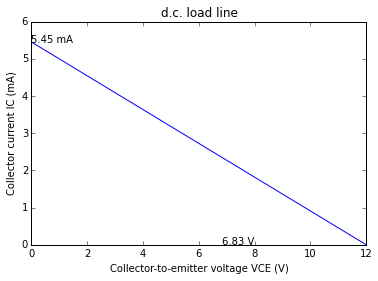## Example 22.9 , Page Number 538¶

In :
import math
import numpy
%matplotlib inline
from matplotlib.pyplot import plot,title,xlabel,ylabel,annotate
#Variables

VCC = 30.0                        #Source voltage (in volts)
RC = 5.0                          #Collector resistance (in kilo-ohm)
RB = 1.5 * 10**3                  #Base Resistance (in kilo-ohm)
beta = 100.0                      #Common-emitter current gain

#Calculation

ICsat = VCC / RC                  #Saturation current (in milli-Ampere)
VCEcutoff = VCC                   #Cut-off voltage (in volts)
IB = VCC / RB                     #Base current (in milli-Ampere)
IC = beta * IB                    #Collector current (in milli-Ampere)
VCE = VCC - IC * RC               #Collector-to-Emitter voltage (in volts)

#Result

print "Operating voltage is ",VCE," V.\nOpearing current is ",IC," mA."

#Graph

x = numpy.linspace(0,30,100)
plot(x,6.0 - 6.0/30.0 * x)
xlabel("Collector-to-emitter voltage VCE (V)")
ylabel("Collector current IC (mA)")
annotate("20 V",xy=(20,0))
annotate("2 mA",xy=(0,2))
annotate("Q",xy=(20,2))

Operating voltage is  20.0  V.
Opearing current is  2.0  mA.

Out:
<matplotlib.text.Annotation at 0x63e64b0>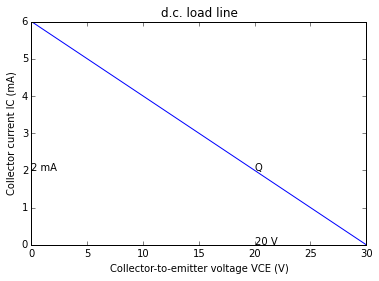## Example 22.10 , Page Number 538¶

In :
import math

#Variables

VCC = 5.0                        #Source voltage (in volts)
RE = 100.0                       #Emitter resistance (in kilo-ohm)
VBE = 0.7                        #Emitter-base Voltage (in volts)

#Calculation

#Case 1 : when VBB = 0.2 V ->OFF
#Case 2: when VBB = 3 V ->ON

#Result

print "When VBB = 0 V , LED is in OFF condition.\nWhen VBB = 3 V , LED is in ON condition."

When VBB = 0 V , LED is in OFF condition.
When VBB = 3 V , LED is in ON condition.


## Example 22.11 , Page Number 539¶

In :
import math

#Variables

VCC = 25.0                        #Source voltage (in volts)
RC = 820.0                        #Collector resistance (in ohm)
RB = 180.0 * 10**3                #Base Resistance (in ohm)
beta = 80.0                       #Common-Emitter current gain
VBE = 0.7                         #Emitter-to-Base Voltage (in volts)
RE = 200.0                        #Emitter resistance (in kilo-ohm)

#Calculation

IC = (VCC -VBE)/(RE + RB / beta)  #Collector current (in milli-Ampere)
VCE = VCC - IC * RC               #Collector-to-Emitter voltage (in volts)
S = 1 + beta                      #Stability factor

#Result

print "Collector current is ",round(IC * 10**3,1)," mA.\nCollector-to-Emitter voltage is ",VCE," V.\nStability factor is ",S,"."

#Stability is not calculated in the book.

Collector current is  9.9  mA.
Collector-to-Emitter voltage is  16.8669387755  V.
Stability factor is  81.0 .


## Example 22.12 , Page Number 540¶

In :
import math
import numpy
%matplotlib inline
from matplotlib.pyplot import plot,title,xlabel,ylabel,annotate

#Variables

VCC = 10.0                        #Source voltage (in volts)
RC = 10.0 * 10**3                 #Collector resistance (in ohm)
RB = 100.0 * 10**3                #Base Resistance (in ohm)
beta = 100.0                      #Common-Emitter current gain
VBE = 0.7                         #Emitter-to-Base Voltage (in volts)

#Calculation

IC = (VCC -VBE)/(RC + RB / beta)  #Collector current (in Ampere)
VCE = VCC - IC * RC               #Collector-to-Emitter voltage (in volts)
ICsat = VCC / RC                  #Saturation current (in milli-Ampere)
VCEcutoff = VCC                   #Cut-off voltage (in volts)

#Result

print "Collector current is ",round(IC * 10**3,3)," mA.\nCollector-to-Emitter voltage is ",round(VCE,2)," V."

#Graph

x = numpy.linspace(0,10,100)
plot(x,6.0 - 6.0/30.0 * x)
xlabel("Collector-to-emitter voltage VCE (V)")
ylabel("Collector current IC (mA)")
annotate("20 V",xy=(20,0))
annotate("2 mA",xy=(0,2))
annotate("Q",xy=(20,2))

Collector current is  0.845  mA.
Collector-to-Emitter voltage is  1.55  V.

Out:
<matplotlib.text.Annotation at 0x71996d0>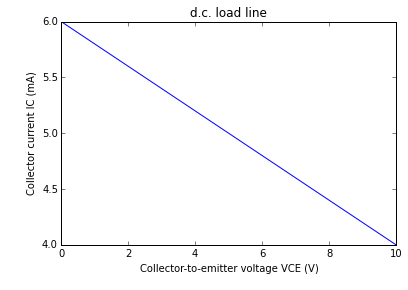## Example 22.13 , Page Number 541¶

In :
import math

#Variables

VCC = 10.0                        #Source voltage (in volts)
RC = 2.0 * 10**3                  #Collector resistance (in ohm)
RB = 100.0 * 10**3                #Base Resistance (in ohm)
beta = 50.0                       #Common-Emitter current gain
VBE = 0.7                         #Emitter-to-Base Voltage (in volts)

#Calculation

IB = (VCC - VBE)/(RB + beta * RC) #Base current (in Ampere)
IC = beta * IB                    #Collector current (in Ampere)
IE = IC                           #Emitter current (in Ampere)
S = 1 + beta                      #Stability factor

#Result

print "IB is ",IB * 10**3," mA.\nIC is ",IC * 10**3," mA.\nIE is ",IE * 10**3," mA."

IB is  0.0465  mA.
IC is  2.325  mA.
IE is  2.325  mA.


## Example 22.14 , Page Number 542¶

In :
import math

#Variables

#When VC = 0 volts
VCC = 9.0                                #Source voltage (in volts)
RB = 220.0                               #Base Resistance (in kilo-ohm)
RC = 3.3                                 #Collector Resistance (in kilo-ohm)
VBE = 0.3                                #Emitter-to-Base voltage (in volts)
beta = 100.0                             #Common emitter current gain

#Calculation

IB = (VCC - VBE)/((RB + beta*RC)* 10**3) #Base current (in Ampere)
IC = beta * IB                           #Collector current (in Ampere)
VCE = VCC - IC * RC * 10**3              #Collector-to-emitter voltage (in volts)
VC = VCE                                 #Collector voltage (in volts)
ICRC = VCC                               #Voltage (in volts)

#When VC = 9 volts
IB1 = 16.0                               #Base current (in micro-Ampere)
IC1 = beta * IB1                         #Collector current (in micro-Ampere)
RC1 = 0                                  #Collector Resistance (in ohm)

#Result

print "In case 1, Collector junction is short circuited.\nIn case 2, Collector resistance is short circuited. "

In case 1, Collector junction is short circuited.
In case 2, Collector resistance is short circuited.


## Example 22.15 , Page Number 542¶

In :
import math

#Variables

VCC = 12.0                               #Source voltage (in volts)
RE = 100.0                               #Emitter Resistance (in ohm)
RC = 3.3                                 #Collector Resistance (in kilo-ohm)
IE = 2.0                                 #Emitter current (in milli-Ampere)
VBE = 0.7                                #Emitter-to-Base Voltage (in volts)
alpha = 0.98                             #Common base current gain
R2 = 20.0                                #Resistance (in kilo-ohm)

#Calculation

IC = alpha * IE                          #Collector current (in milli-Ampere)
VB = VBE + IE * RE * 10**-3              #Base voltage (in volts)
VC = VCC - IC * RC                       #Collector voltage (in volts)
IR2 = VC / (R2)                          #Current through resistance 2 (in milli-Ampere)
IB = IE - IC                             #Base current (in milli-Ampere)
IR1 = IR2 + IB                           #Current through resistance 1 (in milli-Ampere)
R1 = (VC - VB) / IR1                     #Value of the resistance (in kilo-ohm)

#Result

print "The value of R1 is ",round(R1,1)," kilo-ohm."

#Correction to be done in the book about the formula of IR2

The value of R1 is  14.6  kilo-ohm.


## Example 22.16 , Page Number 543¶

In :
import math

#Variables

VCC = 24.0                               #Source voltage (in volts)
RE = 270.0                               #Emitter Resistance (in ohm)
RC = 10.0                                #Collector Resistance (in kilo-ohm)
VBE = 0.7                                #Emitter-to-Base Voltage (in volts)
beta = 45.0                              #Common emitter current gain
VCE = 5.0                                #Collector-to-Emitter voltage (in volts)

#Calculation

IC = (VCC - VCE) / RC                    #Collector current (in milli-Ampere)
RB = ((VCC - VBE) / IC - RC) * beta      #Base Resistance (in kilo-ohm)

#Result

print "Base resistance is ",round(RB,2)," kilo-ohm."

#Calculation mistake in book.

Base resistance is  101.84  kilo-ohm.


## Example 22.17 , Page Number 545¶

In :
import math

#Variables

VCC = 3.0                                #Source voltage (in volts)
RB = 33.0                                #Base Resistance (in kilo-ohm)
RC = 1.8                                 #Collector Resistance (in kilo-ohm)
VBE = 0.7                                #Emitter-to-Base Voltage (in volts)
beta = 90.0                              #Common emitter current gain

#Calculation

IB = (VCC - VBE) / (RB + beta * RC)      #Base current (in milli-Ampere)
IC = beta * IB                           #Collector current (in milli-Ampere)
VCE = VCC -IC * RC                       #Collector-to-emitter voltage (in volts)
S = (1 + beta)/(1 + beta*RC/(RC + RB))   #Stability factor

#Result

print "DC bias current is ",round(IC,2)," mA.\nDC bias voltage is ",round(VCE,1)," V.\nStability factor is ",round(S,1),"."

DC bias current is  1.06  mA.
DC bias voltage is  1.1  V.
Stability factor is  16.1 .


## Example 22.18 , Page Number 546¶

In :
import math

#Variables

VCC = 10.0                                #Source voltage (in volts)
RE = 500.0                                #Emitter Resistance (in ohm)
RC = 1.0                                  #Collector Resistance (in kilo-ohm)
R1 = 10.0                                 #Resistance (in kilo-ohm)
R2 = 5.0                                  #Resistance (in kilo-ohm)
VBE = 0.7                                 #Emitter-to-Base Voltage (in volts)
beta = 100.0                               #Common emitter current gain

#Calculation

VB = VCC * (R2 /(R1 + R2))                #Base voltage (in volts)
VE = VB - VBE                             #Emitter voltage (in volts)
IE = VE / RE                              #Emitter current (in Ampere)
IC = IE                                   #Collector current (in Ampere)
VCE = VCC - IC * (RC * 10**3 + RE)        #Collector-to-Emitter voltage (in volts)

#Result

print "Collector current is ",round(IC * 10**3,2)," mA.\nCollector-to-Emitter voltage is ",VCE," V."

Collector current is  5.27  mA.
Collector-to-Emitter voltage is  2.1  V.


## Example 22.19 , Page Number 547¶

In :
import math

#Variables

VCC = 15.0                                 #Source voltage (in volts)
RE = 2.0                                   #Emitter Resistance (in kilo-ohm)
RC = 1.0                                   #Collector Resistance (in kilo-ohm)
R1 = 10.0                                  #Resistance (in kilo-ohm)
R2 = 5.0                                   #Resistance (in kilo-ohm)
VBE = 0.7                                  #Emitter-to-Base Voltage (in volts)

#Calculation

Vth = VCC * (R2 /(R1 + R2))                #Thevenin's voltage (in volts)
Rth = R1 * R2 / (R1 + R2)                  #Thevenin's equivalent resistance (in kilo-ohm)
IE = (Vth - VBE)/(RE)                      #Emitter current (in milli-Ampere)
VCE = VCC - IE * (RC + RE)                 #Collector-to-Emitter voltage (in volts)

#Result

print "Emitter current is ",IE," mA.\nThe value of collector-to-emitter voltage is ",VCE," V."

Emitter current is  2.15  mA.
The value of collector-to-emitter voltage is  8.55  V.


## Example 22.20 , Page Number 549¶

In :
import math

#Variables

VCC = 12.0                                 #Source voltage (in volts)
RE = 100.0                                 #Emitter Resistance (in ohm)
RC = 1.0                                   #Collector Resistance (in kilo-ohm)
R1 = 25.0                                  #Resistance (in kilo-ohm)
R2 = 5.0                                   #Resistance (in kilo-ohm)
VBE = 0.7                                  #Emitter-to-Base Voltage (in volts)
betamin = 50.0                             #Common emitter current gain (min)
betamax = 150.0                            #Common emitter current gain (max)

#Calculation

Vth = VCC * (R2 /(R1 + R2))                #Thevenin's voltage (in volts)
Rth = R1 * R2 / (R1 + R2) * 10**3          #Thevenin's equivalent resistance (in ohm)
IE1 = (Vth - VBE)/(RE + Rth/betamin)       #Emitter current (in Ampere)
IE2 = (Vth - VBE)/(RE + Rth/betamax)       #Emitter current (in Ampere)
perc_change = (IE2 - IE1) / IE1 * 100      #Percentage change in the value of beta

#Result

print "The percentage change in collector current is ",round(perc_change,1)," %."

The percentage change in collector current is  43.5  %.


## Example 22.21 , Page Number 549¶

In :
import math

#Variables

VCC = 9.0                                  #Source voltage (in volts)
RE = 680.0                                 #Emitter Resistance (in ohm)
RC = 1.0                                   #Collector Resistance (in kilo-ohm)
R1 = 33.0                                  #Resistance (in kilo-ohm)
R2 = 15.0                                  #Resistance (in kilo-ohm)
VBE = 0.7                                  #Emitter-to-Base Voltage (in volts)

#Calculation

VB = VCC * R2 / (R1 + R2)                  #Base voltage (in volts)
VE = VB - VBE                              #Emitter voltage (in volts)
IE = VE / RE                               #Emitter current (in Ampere)
IC = IE                                    #Collector current (in Ampere)
VRC = IC * RC  * 10**3                     #Voltage across collector resistance (in volts)
VC = VCC - VRC                             #Collector voltage (in volts)
VCE = VC - VE                              #Collector-to-emitter voltage (in volts)

#Result

print "Operating point values are IC = ",round(IC * 10**3,1)," mA and VCE = ",round(VCE,3)," V."

Operating point values are IC =  3.1  mA and VCE =  3.781  V.


## Example 22.22 , Page Number 550¶

In :
import math

#Variables

VCC = 5.0                                  #Source voltage (in volts)
RE = 0.3                                   #Emitter Resistance (in kilo-ohm)
IC = 1.0                                   #Collector Current (in milli-Ampere)
beta = 100.0                               #Common emitter current gain
VCE = 2.5                                  #Collector-to-Emitter voltage (in volts)
VBE = 0.7                                  #Emitter-to-Base Voltage (in volts)
ICO = 0                                    #Reverse saturation current (in Ampere)
R2 = 10.0                                  #Resistance (in kilo-ohm)

#Calculation

IE = IC                                    #Emitter current (in milli-Ampere)
RC = (VCC - VCE) / IE - RE                 #Collector resistance (in kilo-ohm)
VE = IE * RE                               #Emitter voltage (in volts)
VB = VE + VBE                              #Base voltage (in volts)
R1 = VCC / VB * R2 - R2                    #Resistance1 (in kilo-ohm)

#Result

print "The value of R1 is ",R1," kilo-ohm and value of RC is ",RC * 10**3," ohm."

The value of R1 is  40.0  kilo-ohm and value of RC is  2200.0  ohm.


## Example 22.23 , Page Number 551¶

In :
import math

#Variables

VCC = 20.0                                 #Source voltage (in volts)
RE = 5.0                                   #Emitter Resistance (in kilo-ohm)
RC = 1.0                                   #Collector Resistance (in kilo-ohm)
R1 = 10.0                                  #Resistance (in kilo-ohm)
R2 = 10.0                                  #Resistance (in kilo-ohm)
VBE = 0.7                                  #Emitter-to-Base Voltage (in volts)

#Calculation

VB = VCC * R2 / (R1 + R2)                  #Voltage (in volts)
VE = VB - VBE                              #Emitter voltage (in volts)
IE = VE / RE                               #Emitter current (in milli-Ampere)
IC = IE                                    #Collector current (in milli-Ampere)
VCE = VCC - IC * RC                        #Collector-to-emitter voltage (in volts)
VC = VCE + VE                              #Collector potential (in volts)

#Result

print "Emitter current is ",IE," mA.\nValue of VCE is ",VCE," V.\nValue of collector potential is ",VC," V."

#VC is not calculated in the book.

Emitter current is  1.86  mA.
Value of VCE is  18.14  V.
Value of collector potential is  27.44  V.


## Example 22.24 , Page Number 552¶

In :
import math

#Variables

VCC = 8.0                           #Source voltage (in volts)
VRC = 0.5                           #Voltage across collector resistance (in volts)
RC = 800.0                          #Collector resistance (in ohm)
alpha = 0.96                        #common base current gain

#Calculation

VCE = VCC - VRC                     #Collector-to-emitter voltage (in volts)
IC = VRC / RC                       #Collector current (in milli-Ampere)
IE = IC / alpha                     #Emitter current (in milli-Ampere)
IB = IE - IC                        #Base current (in milli-Ampere)

#Result

print "Collector-to-Emitter VCE is ",VCE," V.\nBase current is ",round(IB * 10**3,3)," mA."

Collector-to-Emitter VCE is  7.5  V.
Base current is  0.026  mA.


## Example 22.27 , Page Number 554¶

In :
import math

#Variables

beta = 50.0                          #Common emitter current gain
VBE = 0.7                            #Emitter-to-Base Voltage (in volts)
VCC = 22.5                           #Source voltage (in volts)
RC = 5.6                             #Collector Resistance (in kilo-ohm)
VCE = 12.0                           #Collector-to-emitter voltage (in volts)
IC = 1.5                             #Collector current (in milli-Ampere)
#Stability factor (S) <= 3.
S = 3

#Calculation

RE = (VCC - VCE)/IC - RC             #Emitter resistance (in kilo-ohm)
Rth = (4375 - (1.4 * 10**3))*10**-3  #Thevenin's Equivalent Resistance (in ohm)
R2 = 0.1 * beta * RE                 #Resistance (in kilo-ohm)
R1 = (R2 - Rth)**-1 * R2 *Rth        #Resistance 1 (in kilo-ohm)

#Result

print "Value of RE is ",RE ," kilo-ohm.\nValue of R1 is ",round(R1,1)," kilo-ohm.\nValue of R2 is ",R2," kilo-ohm."

Value of RE is  1.4  kilo-ohm.
Value of R1 is  5.2  kilo-ohm.
Value of R2 is  7.0  kilo-ohm.


## Example 22.28 , Page Number 556¶

In :
import math

#Variables

VEE = 10.0                           #Emitter Bias Voltage (in volts)
VCC = 10.0                           #Source voltage (in volts)
RC = 1.0                             #Collector Resistance (in kilo-ohm)
RE = 5.0                             #Emitter Resistance (in kilo-ohm)
RB = 50.0                            #Base Resistance (in kilo-ohm)
VBE = 0.7                            #Emitter-to-Base Voltage (in volts)

#Calculation

VE = -VBE                            #Emitter voltage (in volts)
IE = (VEE - VBE)/ RE                 #Emitter current (in milli-Ampere)
IC = IE                              #Collector current (in milli-Ampere)
VC = VCC - IC * RC                   #Collector voltage (in volts)
VCE = VC - VE                        #Collector-to-Emitter voltage (in volts)

#Result

print "The value of emitter current is ",IE," mA.\nTHe value of collector current is ",IC," mA.\nThe value of collector-to-emitter voltage is ",VCE," V."

The value of emitter current is  1.86  mA.
THe value of collector current is  1.86  mA.
The value of collector-to-emitter voltage is  8.84  V.


## Example 22.29 , Page Number 557¶

In :
import math

#Variables

VEE = 20.0                           #Emitter Bias Voltage (in volts)
VCC = 20.0                           #Source voltage (in volts)
RC = 5.0                             #Collector Resistance (in kilo-ohm)
RE = 10.0                            #Emitter Resistance (in kilo-ohm)
RB = 10.0                            #Base Resistance (in kilo-ohm)
VE = -0.7                            #Emitter Voltage (in volts)
betamin = 50.0                       #Common emitter current gain minimum
betamax = 100.0                      #Common emitter current gain maximum
VE1 = -0.6                           #Emitter Voltage1 (in volts)
VBE = 0.7                            #Emitter-to-base voltage (in volts)
VBE1 = 0.6                           #New emitter-to-base voltage (in volts)

#Calculation

IE = (VEE - VBE)/(RE + RB / betamin) #Emitter current (in milli-Ampere)
IC = IE                              #Collector current (in milli-Ampere)
VC = VCC - IC * RC                   #Collector voltage (in volts)
VCE = VC - VE                        #Collector-to-emitter voltage (in volts)
IE1 = (VEE - VBE1)/(RE + RB/betamax) #Emitter current 1 (in milli-Ampere)
IC1 = IE1                            #Collector current 1 (in milli-Ampere)
VC1 = VCC - IC1 * RC                 #Collector voltage 1 (in volts)
VCE1 = VC1 - VE1                     #Collector-to-emitter voltage 1 (in volts)
dIC = (IC1 - IC) / IC                #Change in collector current
dVCE = (VCE - VCE1) / VCE            #Change in collector to emitter voltage

#Result

print "The change is collector current is ",round(dIC,5)* 100,"%.\nThe change in collector to emitter voltage is ",100*round(dVCE,4),"%."

#Slight changes due to higher precision.

The change is collector current is  1.513 %.
The change in collector to emitter voltage is  2.16 %.


## Example 22.30 , Page Number 561¶

In :
import math

#Variables

VCC = 12.0                                  #Source voltage (in volts)
RE = 1.0                                    #Emitter Resistance (in kilo-ohm)
RC = 2.0                                    #Collector Resistance (in kilo-ohm)
R1 = 100.0                                  #Resistance (in kilo-ohm)
R2 = 20.0                                   #Resistance (in kilo-ohm)
VBE = -0.2                                  #Emitter-to-Base Voltage (in volts)
beta = 100.0                                #Common emitter current gain

#Calculation

VB = -VCC * R2 / (R1 + R2)                  #Base voltage (in volts)
VE = VB - VBE                               #Emitter voltage (in volts)
IE = -VE / RE                               #Emitter current (in milli-Ampere)
IC = IE                                     #Collector current (in milli-Ampere)
VC = -(VCC - IC * RC)                       #Collector voltage (in volts)
VCE = VC - VE                               #Collector-to-emitter voltage (in volts)

#Result

print "Base voltage is ",VB," V.\nEmitter voltage is ",VE," V.\nCollector voltage is ",VC," V.\nCollector current is ",IC," mA.\nEmitter current is ",IE," mA.\nCollector-to-emitter voltage is ",VCE," V."

#Formula of IE and VB is given wrong in the book.

Base voltage is  -2.0  V.
Emitter voltage is  -1.8  V.
Collector voltage is  -8.4  V.
Collector current is  1.8  mA.
Emitter current is  1.8  mA.
Collector-to-emitter voltage is  -6.6  V.


## Example 22.31 , Page Number 561¶

In :
import math

#Variables

VCC = 10.0                                   #Source voltage (in volts)
RE = 2.0                                     #Emitter Resistance (in kilo-ohm)
RC = 10.0                                    #Collector Resistance (in kilo-ohm)
R1 = 16.0                                    #Resistance (in kilo-ohm)
R2 = 4.0                                     #Resistance (in kilo-ohm)
VBE = 0.7                                    #Emitter-to-Base Voltage (in volts)
beta = 100.0                                 #Common emitter current gain

#Calculation

VB = VCC * R2 / (R1 + R2)                    #Base voltage (in volts)
VE = VB - VBE                                #Emitter voltage (in volts)

#Result

print "The value of base voltage is ",VB," V.\nThe value of emitter voltage is ",VE," V."

The value of base voltage is  2.0  V.
The value of emitter voltage is  1.3  V.


## Example 22.32 , Page Number 562¶

In :
import math

#Variables

VCC = 4.5                                    #Source voltage (in volts)
RE = 0.27                                    #Emitter Resistance (in kilo-ohm)
RC = 1.5                                     #Collector Resistance (in kilo-ohm)
R1 = 27.0                                    #Resistance (in kilo-ohm)
R2 = 2.7                                     #Resistance (in kilo-ohm)
VBE = 0.3                                    #Emitter-to-Base Voltage for germanium (in volts)
beta = 44.0                                  #Common emitter current gain

#Calculation

VB = - VCC * R2 / (R1 + R2)                  #Base voltage (in volts)
VE = VB - (-VBE)                             #Emitter voltage (in volts)
IE = VE / RE                                 #Emitter current (in milli-Ampere)
IC = IE                                      #Collector current (in milli-Ampere)
VRC = -IC * RC                               #Voltage across collector resistance (in volts)
VC = -(VCC - VRC)                            #Collector voltage (in volts)
VCE = -(-VC - (-VE))                         #Collector-to-emitter voltage (in volts)

#Result

print "The operating point values are IC = ",round(-IC,3)," mA and VCE = ",round(VCE,2)," V."

The operating point values are IC =  0.404  mA and VCE =  -3.78  V.